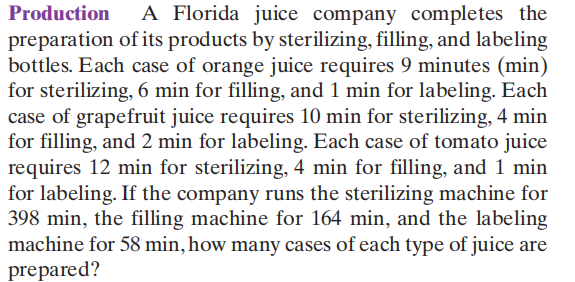### ¿Todavía tienes preguntas de matemáticas?

Pregunte a nuestros tutores expertos
Algebra
PreguntaA Florida juice company completes the preparation of its products by sterilizing, filling, and labeling bottles. Each case of orange juice requires $$9$$ minutes (min) for sterilizing, $$6$$ min for filling, and $$1$$ min for labeling. Each case of grapefruit juice requires $$10$$ min for sterilizing, $$4$$ min for filling, and $$2$$ min for labeling. Each case of tomato juice requires $$12$$ min for sterilizing, $$4$$ min for filling, and $$1$$ min for labeling. If the company runs the sterilizing machine for

$$398$$ min, the filling machine for $$164$$ min, and the labeling machine for $$58$$ min, how many cases of each type of juice are prepared?

$$x = 20$$ cases of orange juice,
$$y = 12$$ cases of tomato juice,
$$z = 6$$ cases of pineapple juice.